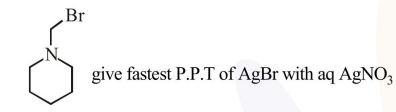# Which of the following compounds will form the precipitate with aq.

Question:

Which of the following compounds will form the precipitate with aq. $\mathrm{AgNO}_{3}$ solution most readily ?

1.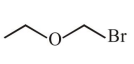2.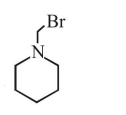3.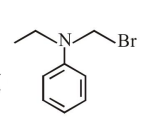4.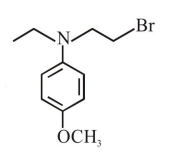Correct Option: , 2

Solution:

$\mathrm{R}-\mathrm{x}+\mathrm{aq} \cdot \mathrm{AgNO}_{3} \stackrel{\text { R.D. }}{\longrightarrow} \mathrm{R}^{\oplus}+\underset{(\mathrm{PPT})}{\mathrm{Agx}}$

So rate of P.P.T formation of $\mathrm{Agx}$ depend's on stability of carbocation $\left(\mathrm{R}^{+}\right)$

In given question formed carbocation will be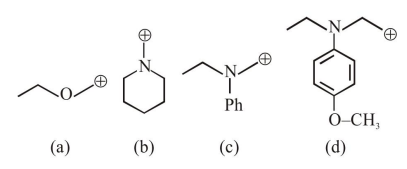Most stable carbocation is (b) so